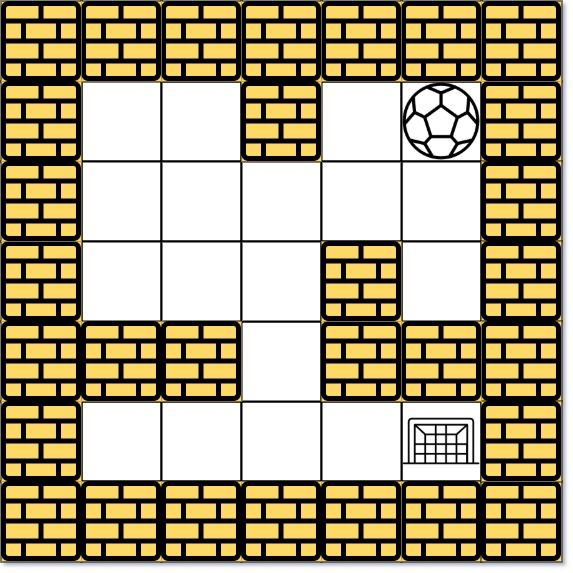### LeetCode• ㊗️
• 大家
• offer
• 多多！

## Problem

There is a ball in a maze with empty spaces (represented as 0) and walls (represented as 1). The ball can go through the empty spaces by rolling up, down, left or right, but it won’t stop rolling until hitting a wall. When the ball stops, it could choose the next direction.

Given the m x n maze, the ball’s start position and the destination, where start = [startrow, startcol] and destination = [destinationrow, destinationcol], return true if the ball can stop at the destination, otherwise return false.

You may assume that the borders of the maze are all walls (see examples).

Example 1:Input: maze = [[0,0,1,0,0],[0,0,0,0,0],[0,0,0,1,0],[1,1,0,1,1],[0,0,0,0,0]], start = [0,4], destination = [4,4]
Output: true
Explanation: One possible way is : left -> down -> left -> down -> right -> down -> right.


Example 2:

Input: maze = [[0,0,1,0,0],[0,0,0,0,0],[0,0,0,1,0],[1,1,0,1,1],[0,0,0,0,0]], start = [0,4], destination = [3,2]
Output: false
Explanation: There is no way for the ball to stop at the destination. Notice that you can pass through the destination but you cannot stop there.


Example 3:

Input: maze = [[0,0,0,0,0],[1,1,0,0,1],[0,0,0,0,0],[0,1,0,0,1],[0,1,0,0,0]], start = [4,3], destination = [0,1]
Output: false


Constraints:

• m == maze.length
• n == maze[i].length
• 1 <= m, n <= 100
• maze[i][j] is 0 or 1.
• start.length == 2
• destination.length == 2
• 0 <= start_row, destination_row <= m
• 0 <= start_col, destination_col <= n
• Both the ball and the destination exist in an empty space, and they will not be in the same position initially.
• The maze contains at least 2 empty spaces.

## Code

class Solution {
public boolean hasPath(int[][] maze, int[] start, int[] destination) {
int m = maze.length;
int n = maze.length;

boolean[][] visited = new boolean[m][n];
int[][] dirs = {{0, 1}, {0, -1}, {-1, 0}, {1, 0}};;

Queue <int[]> queue = new LinkedList <> ();
// 使用二维数组标记visited
visited[start][start] = true;

while (!queue.isEmpty()) {
int[] curr = queue.poll();

if (curr == destination && curr == destination){
return true;
}

for (int[] dir: dirs) {
int x = curr;
int y = curr;

// 一直走到墙或者到头
while (x + dir>= 0 &&
y + dir >= 0 &&
x + dir < m &&
y + dir < n &&
maze[x + dir][y + dir] == 0) {
x += dir;
y += dir;
}

// 上一步是否访问过
if (!visited[x][y]) {
visited[x][y] = true;
}
}
}

return false;
}
}

class Solution {
int[][] dirs={{0, 1}, {0, -1}, {-1, 0}, {1, 0}};

public boolean hasPath(int[][] maze, int[] start, int[] destination) {
return helper(maze, start, destination, new boolean[maze.length][maze.length]);
}

private boolean helper(int[][] maze, int[] curr, int[] dest, boolean[][] visited) {
if(curr == dest && curr == dest) return true;

if(visited[curr][curr]) return false;

visited[curr][curr] = true;

for(int[] dir : dirs) {
int x = curr;
int y = curr;

while(x + dir >= 0 &&
x + dir < maze.length &&
y + dir >= 0 &&
y + dir < maze.length &&
maze[x + dir][y + dir] != 1) {
x += dir;
y += dir;
}

if(helper(maze, new int[]{x, y}, dest, visited)){
return true;
}
}

return false;
}
}